Start typing, then use the up and down arrows to select an option from the list.
5:20 minutes
Problem 105
Textbook Question

# The ionization energy of an atom can be measured by photo-electron spectroscopy, in which light of wavelength l is directed at an atom, causing an electron to be ejected. The kinetic energy of the ejected electron 1EK2 is measured by determining its velocity, v since EK = 1/2 mv2. The Ei is then calculated using the relationship that the energy of the inci-dent light equals the sum of Ei plus EK. (a) What is the ionization energy of rubidium atoms in kilo-joules per mole if light with l = 58.4 nm produces elec-trons with a velocity of 2.450 * 106m/s? (The mass of an electron is 9.109 * 10-31 kg.)Verified Solution
This video solution was recommended by our tutors as helpful for the problem above.
222views

### Watch next

Master Periodic Trend: Ionization Energy with a bite sized video explanation from Jules Bruno

Start learning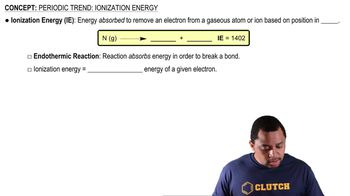01:19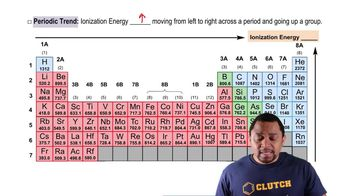01:08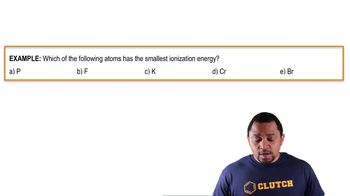00:40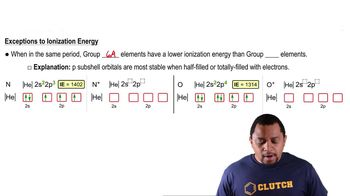01:32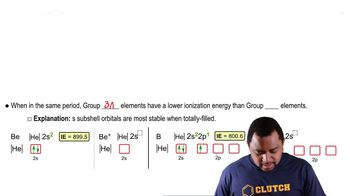01:25